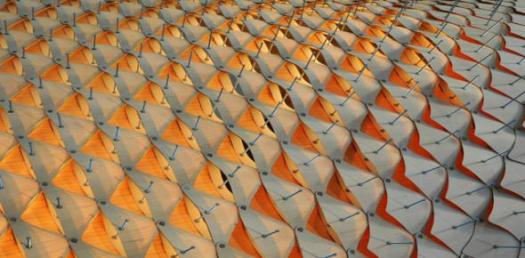# Lattice Trivia Quiz

10 Questions | Total Attempts: 110SettingsLattice is an interwoven structure or pattern. It is a repeated three-dimensional arrangement of atoms, ions, or molecules in a metal or other crystalline solid in a regular manner. Try this quiz to test your knowledge on Lattices.

Related Topics
• 1.
Which of these is true for Lattices?
• A.

They are fixed

• B.

They are partially ordered sets

• C.

They show dimensional property

• D.

They are partly unclear

• 2.
Which other subject is lattice studied?
• A.

Commerce

• B.

History

• C.

Physics

• D.

Government

• 3.
What is the term given to a poset which all its sunset have a join and a meet?
• A.

Complete Lattice

• B.

Joint lattice

• C.

Interpolated lattice

• D.

Bond Lattice

• 4.
What does the symbol ∨ mean?
• A.

Join

• B.

Meet

• C.

Joint

• D.

Connection

• 5.
What does the ∧ symbol denote?
• A.

Join

• B.

Meet

• C.

Joint

• D.

Bond

• 6.
Which of these is the only defining identity peculiar to lattice Theory?
• A.

Idempotent law

• B.

Bounded law

• C.

Absorbtion law

• D.

Rational law

• 7.
Which of these is correct for idempotent law?
• A.

A ∨ a = a

• B.

A ∧ b = b ∧ a

• C.

A ∧ (a ∨ b) = a

• D.

A ∨ 0 = a

• 8.
Which of these play an essential role in universal algebra?
• A.

Arithmetic interpretation of lattice

• B.

Idempotent  law

• C.

Algebraic interpretation of lattice

• D.

Absorbent law

• 9.
How can the collection of all subset of a set be ordered?
• A.

Union inclusion

• B.

Intersection

• C.

Subset inclusion

• D.

Universal inclusion

• 10.
Which of these is true for a complete Lattice?
• A.

Crystal identity is common

• B.

All complete Lattices are bounded lattices

• C.

Idempodent law is of no effect

• D.

It has a low and high meet and Join